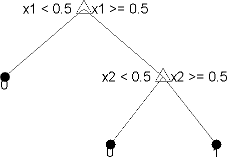## Decision Trees

Decision trees, or classification trees and regression trees, predict responses to data. To predict a response, follow the decisions in the tree from the root (beginning) node down to a leaf node. The leaf node contains the response. Classification trees give responses that are nominal, such as `'true'` or `'false'`. Regression trees give numeric responses.

Statistics and Machine Learning Toolbox™ trees are binary. Each step in a prediction involves checking the value of one predictor (variable). For example, here is a simple classification tree:This tree predicts classifications based on two predictors, `x1` and `x2`. To predict, start at the top node, represented by a triangle (Δ). The first decision is whether `x1` is smaller than `0.5`. If so, follow the left branch, and see that the tree classifies the data as type `0`.

If, however, `x1` exceeds `0.5`, then follow the right branch to the lower-right triangle node. Here the tree asks if `x2` is smaller than `0.5`. If so, then follow the left branch to see that the tree classifies the data as type `0`. If not, then follow the right branch to see that the tree classifies the data as type `1`.

To learn how to prepare your data for classification or regression using decision trees, see Steps in Supervised Learning.

### Train Classification Tree

This example shows how to train a classification tree.

Create a classification tree using the entire `ionosphere` data set.

```load ionosphere % Contains X and Y variables Mdl = fitctree(X,Y)```
```Mdl = ClassificationTree ResponseName: 'Y' CategoricalPredictors: [] ClassNames: {'b' 'g'} ScoreTransform: 'none' NumObservations: 351 Properties, Methods ```

### Train Regression Tree

This example shows how to train a regression tree.

Create a regression tree using all observation in the `carsmall` data set. Consider the `Horsepower` and `Weight` vectors as predictor variables, and the `MPG` vector as the response.

```load carsmall % Contains Horsepower, Weight, MPG X = [Horsepower Weight]; Mdl = fitrtree(X,MPG)```
```Mdl = RegressionTree ResponseName: 'Y' CategoricalPredictors: [] ResponseTransform: 'none' NumObservations: 94 Properties, Methods ```

 Breiman, L., J. H. Friedman, R. A. Olshen, and C. J. Stone. Classification and Regression Trees. Boca Raton, FL: Chapman & Hall, 1984.